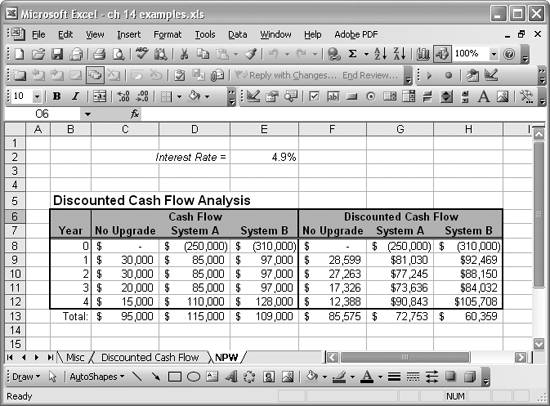# Assessing Net Present Worth

Problem

You're considering certain equipment upgrades in your lab and you want to perform a net present worth analysis of the projected cash flow streams from these upgrades to determine which option presents the best alternative.

Solution

You can use the same techniques discussed in Recipe 14.6 to discount the projected cash flow streams in terms of present values. The option with the highest net present value is the best choice.

Discussion

Consider this example: you're currently running a numerical simulation laboratory that uses an aging supercomputer to run your simulations. You've forecast cash flows for your simulation services over the next five years. Now you're considering purchasing one of two candidate cluster-based systems that will boost your forecast cash flow over the next five years. These new clusters cost money, though, so you're not sure which one, if either, offers the best choice economically speaking. You need to decide whether to purchase system A, system B, or nothing at all.

Let's further assume that system A costs \$250,000 and system B, which is somewhat more powerful than system A, costs \$310,000. Also, at the end of the fifth year you can sell either system for salvage at 10% of its original cost.

You've worked out cash flow forecasts for these two options as shown in Figure 14-2.

The No Upgrade option means you don't upgrade your system, but instead continue offering services over the next five years using your current system. In this case, your cash flow is projected to decrease somewhat over the five-year period due to anticipated increases in operating and maintenance costs. Forecast cash flows for the system A option are expected to be fairly high, and the cash flow in the fifth year includes the 10% salvage value. Similarly, system B is expected to generate more cash flow due to its higher performance capabilities. The fifth-year cash flow value for system B also includes the 10% salvage value.

Examination of the net cash flow values shown in the Total row for the first three cash flow columns in the table in Figure 14-2 gives the impression that upgrading to system A offers the best choice. This is before discounting and figuring out the net present value of each option.

Figure 14-2. Net present worth exampleDiscounting these cash flows uses the same formulas shown in Recipe 14.6. The formulas in the last three cash flow columns, the discounted columns, are of the form =PV(\$E\$2,\$B9,0,-C9,0), where the present value function, PV, is used to compute the present value of each year's cash flow amount for each option.

Net present values are represented by the totals for the last three discounted cash flow columns. Examining these totals reveals that purchasing system A is not the best option as originally thought. Instead the No Upgrade option offers the best alternative at this time. Upon viewing results like this, you might be inclined to hold off on your upgrade until new system prices come down a bit, in which case the net present worth analysis might yield different results. Other factors to consider include fluctuations in interest rates, as well as the declining cash flow of the old system as it ages further.

Excel offers a built-in function called NPV that computes the net present value of a series of cash flows given a discount rate (interest rate). Its syntax is = NPV(rate, values), where rate is the interest rate and values is a cell range or series of values separated by commas representing the cash flow series. You can use NPV to compute net present values for the cash flows shown in the first three columns of the table in Figure 14-2. Doing so will save you the trouble of constructing the discounted cash flow tables and using the PV function.

For example, to compute the net present value of the No Upgrade option, use the formula =C8+NPV(E2,C9:C12). Notice that this formula discounts only the cash flow values for the second through fifth years. The first year, year 0, is then added to the discounted cash flow series to arrive at the net present value. Similarly, the formulas =D8+NPV(E2,D9:D12) and =E8+NPV(E2,E9:E12) compute the net present values for the system A and system B options, respectively. These three formulas yield the same net present value results computed using the PV function.

Check out Recipe 14.9 to learn how to compute internal rates of return for the options in this example.Excel Scientific and Engineering Cookbook (Cookbooks (OReilly))
ISBN: 0596008791
EAN: 2147483647
Year: N/A
Pages: 206
Authors: David M Bourg